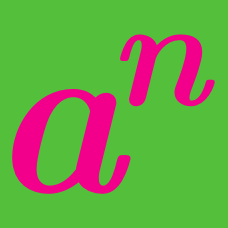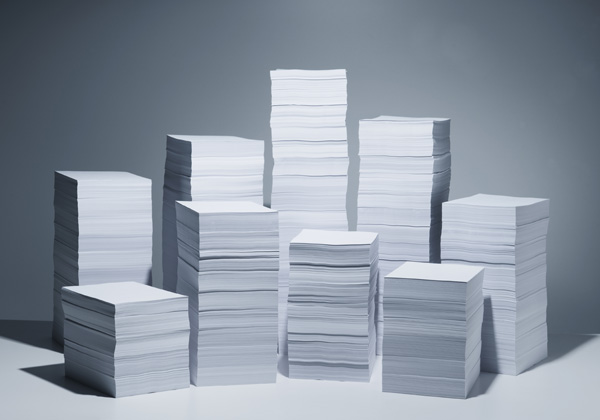Algebra

Exponential Functions: Level 3 Challenges

$\Huge \sqrt{\color{#D61F06}9^{\color{#20A900}{16} \color{#3D99F6}{x^2}}}$

Which of the following is equal to the above expression?

$\large A = 2^{5555}, \qquad B = 3^{3333}, \qquad C = 6^{2222}$

What is the order of these numbers from smallest to largest?

If $2^x = 3^y = 6,$ then which of the following statements is true?

Find the sum of all integer solutions to the equation

$\large (x^2 - 3x + 1)^{x + 1} = 1.$If you have a piece of paper that is $0.1\text{ mm}$ thick, then how many times will you have to fold it in half in order for it to become tall enough to reach the moon?

Note: The distance from the earth to the moon is $384400\text{ km}$.

You have to round off the answer that you are getting to get the answer that you will enter in the answer box.

×